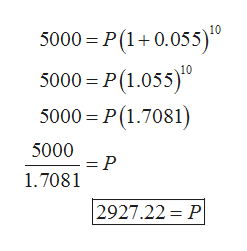# Section 3.2 (27)You want to buy a 10-year bond with a maturity value of \$5000, and you wish to get a return of5.5%  annually. How much will you pay?

Question
79 views

Section 3.2 (27)

You want to buy a 10-year bond with a maturity value of \$5000, and you wish to get a return of5.5%  annually. How much will you pay?

check_circle

Step 1

According to the given information

Maturity value(V) = \$5000

Rate of interest (r) = 5.5% = 0.055

Time (t) = 10 years

It is required to calculate the amount to pay that is principal (P)

The formula used is:

Step 2

Now plug in the...help_outlineImage Transcriptionclose10 5000 = P(1+0.055)" 5000 = P(1.055)" 5000 = P(1.7081) 5000 1.7081 2927.22 = P fullscreen

### Want to see the full answer?

See Solution

#### Want to see this answer and more?

Solutions are written by subject experts who are available 24/7. Questions are typically answered within 1 hour.*

See Solution
*Response times may vary by subject and question.
Tagged in

### Math